Function Repository Resource:

# SectionContourPlot

Give a contour plot of a function together with the projections onto the xy-plane of the given sections of the plot

Contributed by: Dennis M Schneider
 ResourceFunction["SectionContourPlot"][f,{x,xmin,xmax},{y,ymin,ymax},opts] plots the projections onto the xy-plane of the sections of the surface specified with the options "XSection","YSection","ZSection","VSection" and "OSection". ResourceFunction["SectionContourPlot"][f,{x,xmin,xmax},{y,ymin,ymax},{{gx,gy},…},{t,tmin,tmax}] plots the projections onto the xy-plane of the sections of the surface determined by the cylinders described parametrically by {{gx,gy},…},{t,tmin,tmax}, together with the projections onto the xy-plane of the other sections specified with the options "XSection","YSection","ZSection","VSection" and "OSection".

## Details and Options

ResourceFunction["SectionContourPlot"] has the same relationship to the resource function SectionPlot3D that ContourPlot has to Plot3D.
ResourceFunction["SectionContourPlot"] has the same options as ContourPlot with the following additions and changes:
 "DrawPoint" {} a list of points to be included in the plot "DrawPointStyle" {{Red,PointSize[Large]}} styles to be applied to the points specified with the option "DrawPoint" "DrawGradient" {} a list of points at which to draw the gradient "GradientStyle {{Black,PointSize[Medium]}} styles to be applied to the gradient vectors "OSection" {} draws the projection onto the xy-plane of an oblique section determined by functions of the form z=f[x],z=g[y] or z=h[x,y] "OSectionStyle" {{Red,Thickness[.01]}} styles to be applied to the oblique sections "PlotPointsSection" 75 specifies how many initial sample points to use in plotting sections "SectionStyle" {Red,Thickness[.01]} styles to be applied to sections defined parametrically by {gx,gy} "VSection" {} draws the projection onto the xy-plane of vertical sections for a function or list of functions of the form y=f[x] or x=g[y] "VSectionStyle" {Red,Thickness[.01]} styles to be applied to the vertical sections "XSection" {} draws the projection onto the xy-plane of vertical sections parallel to the yz-plane determined by the value or list of values of x "XSectionStyle" {Red,Thickness[.01]} styles to be applied to the x-sections "YSection" {} draws the projection onto the xy-plane of vertical sections parallel to the xz-plane determined by the value or list of values of y "YSectionStyle" {Red,Thickness[.01]} styles to be applied to the y-sections "ZSection" {} draws the projection onto the xy-plane of horizontal sections parallel to the xy-plane determined by the value or list of values of z "ZSectionStyle" {Red,Thickness[.01]} styles to be applied to the z-sections

## Examples

### Basic Examples (2)

A contour plot with projections of an x-section and an oblique section:

 In:=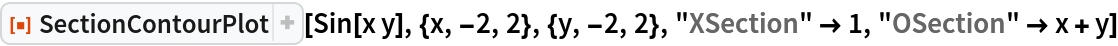Out=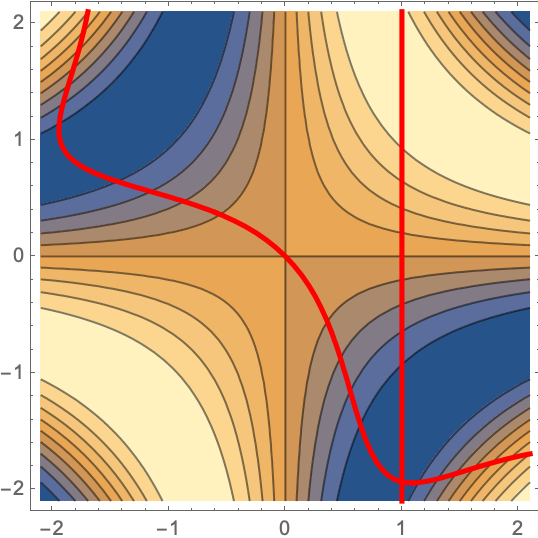Display the resource function SectionPlot3D and SectionContourPlot as a row:

 In:=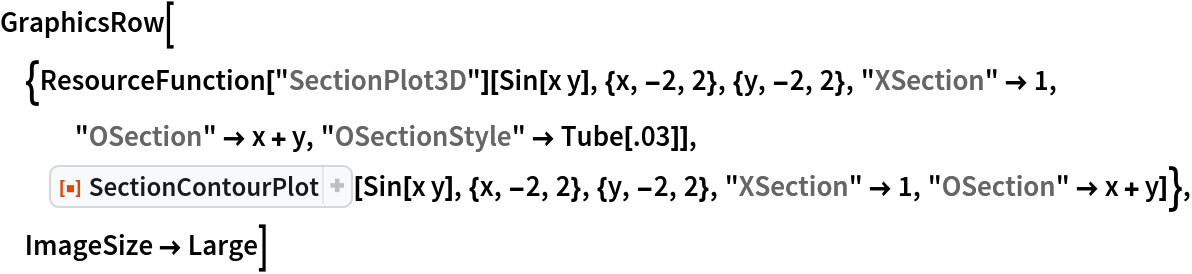Out=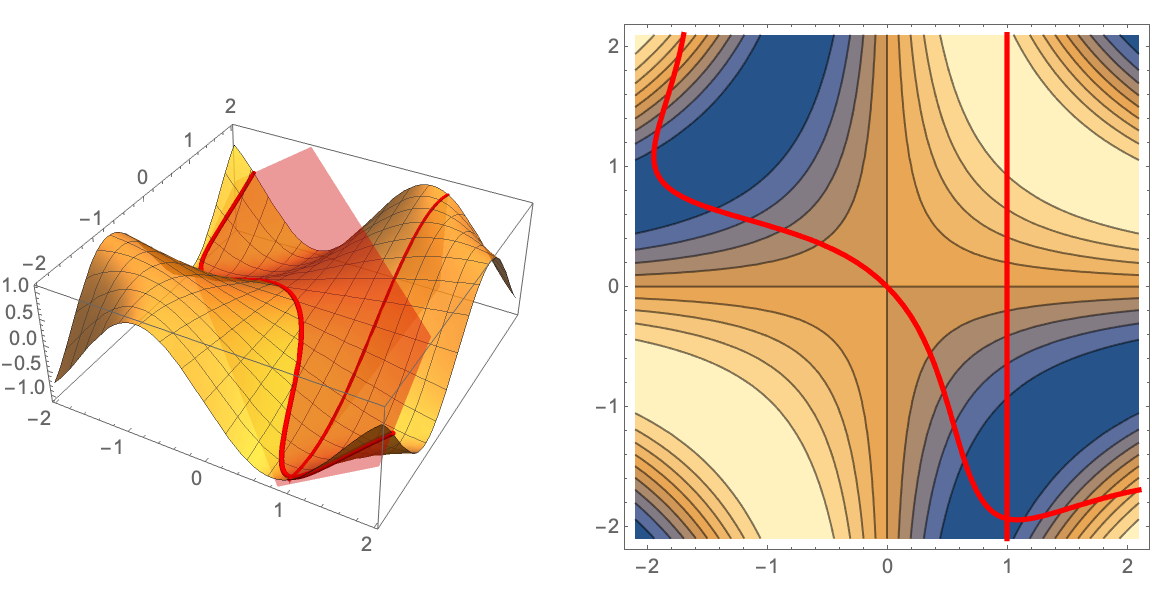A contour plot with the projection onto the xy-plane of a cylinder and a z-section:

 In:=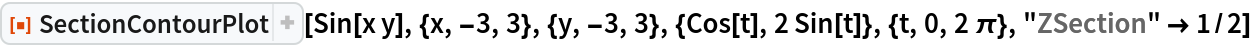Out=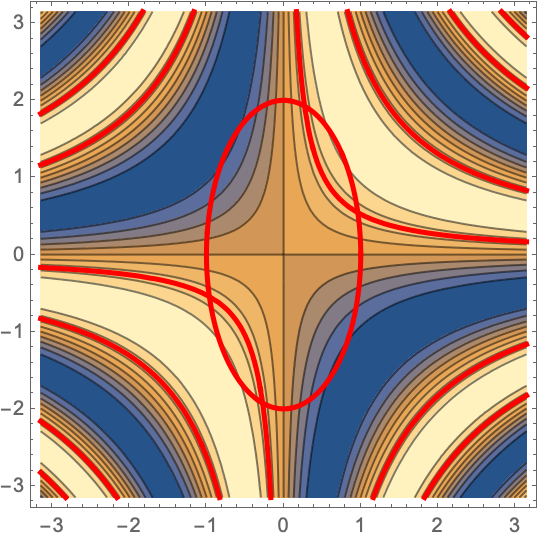Display the resource function SectionPlot3D and SectionContourPlot as a row:

 In:=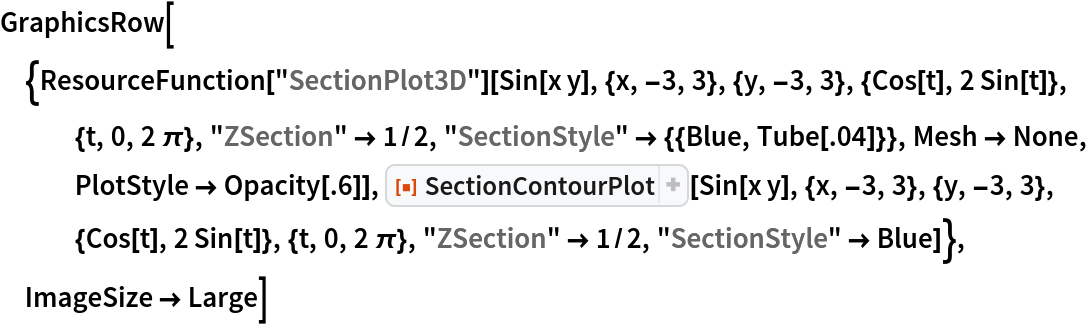Out=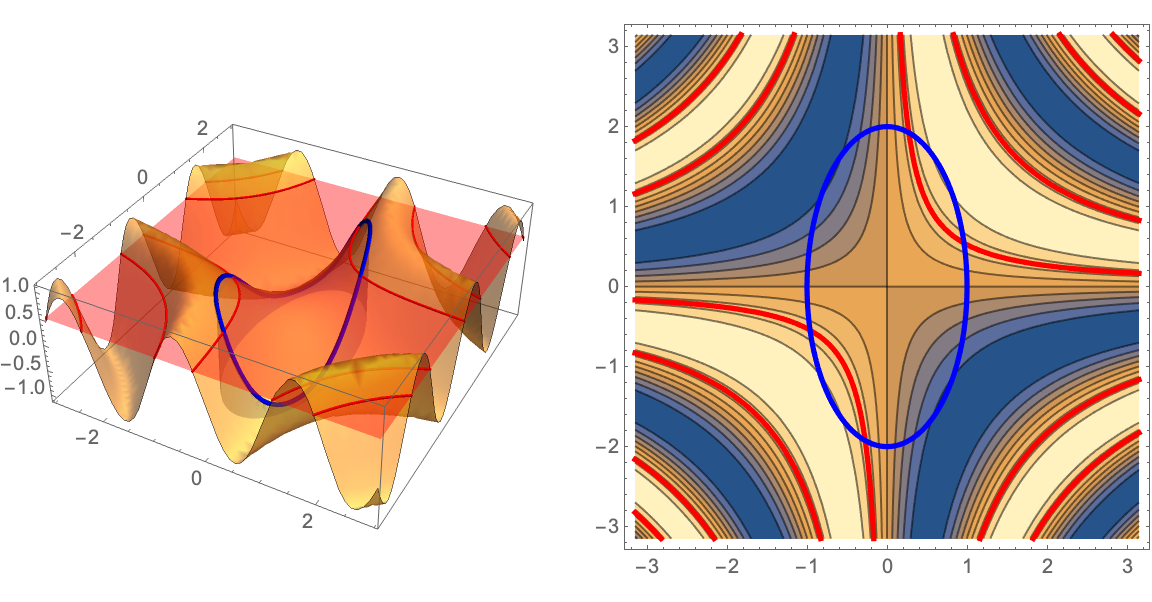View the resource function SectionPlot3D directly from above to illustrate the projection of the sections onto the xy-plane:

 In:=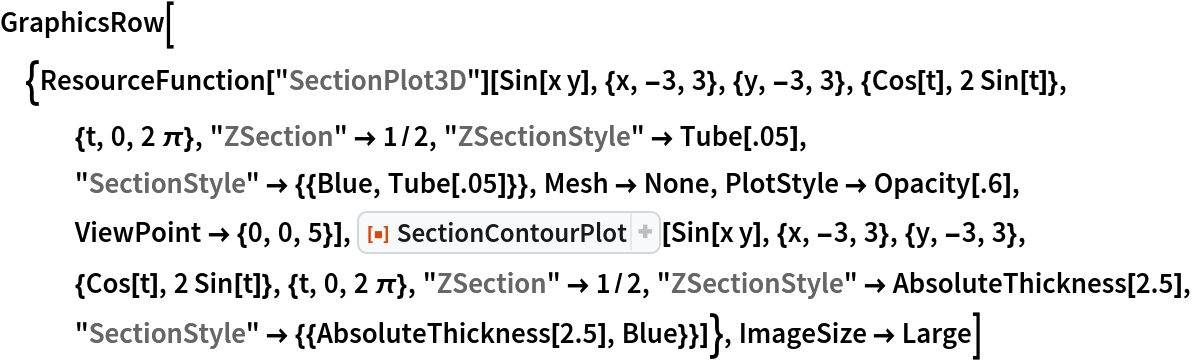Out=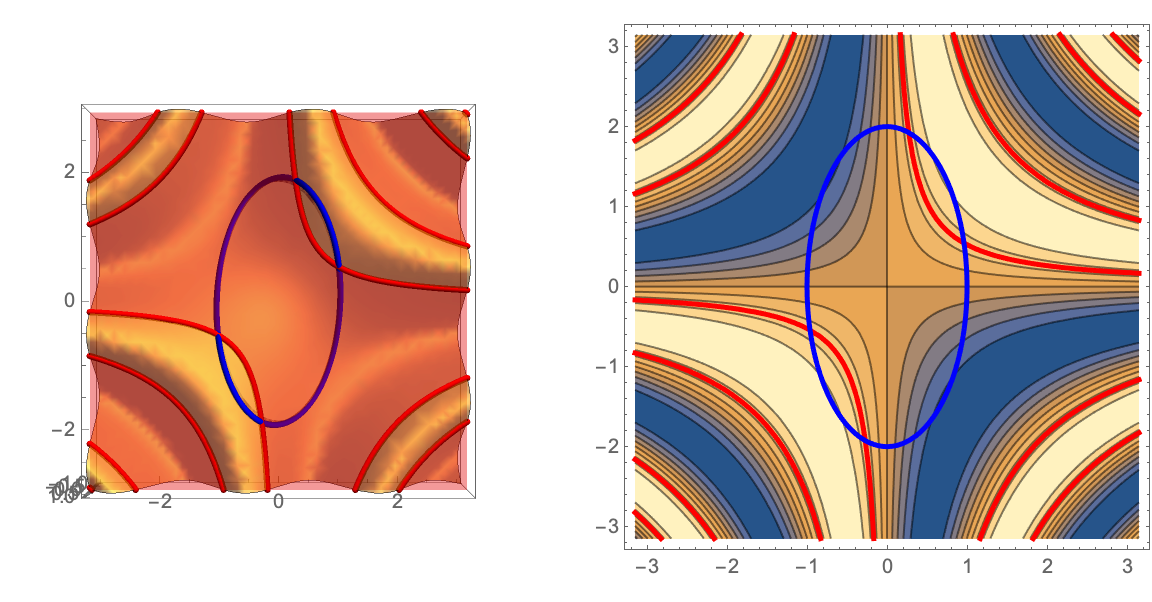### Options (9)

#### DrawPoint and DrawPointStyle (1)

Add points to the contour plot and add styles to the points:

 In:=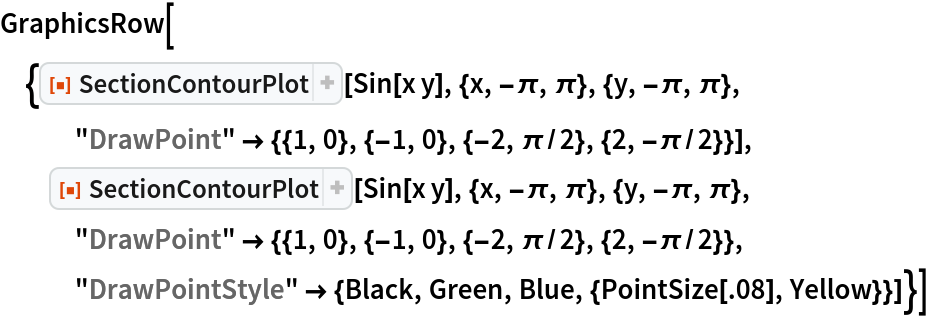Out=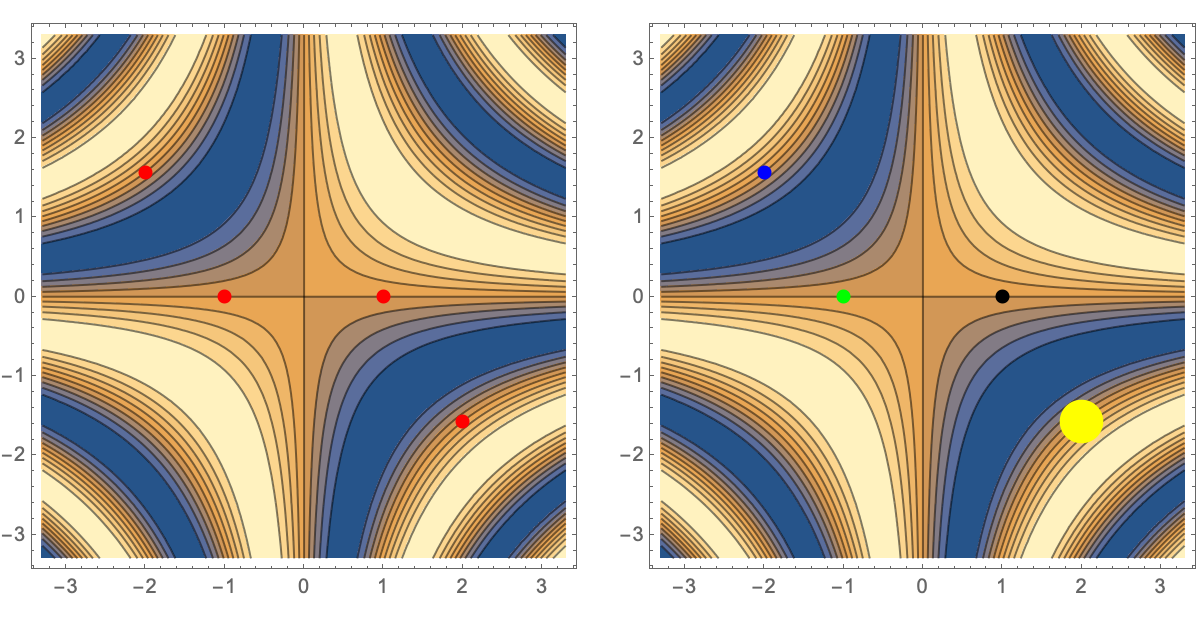Plot the gradient of the function at several points and apply styles to the gradients:

 In:=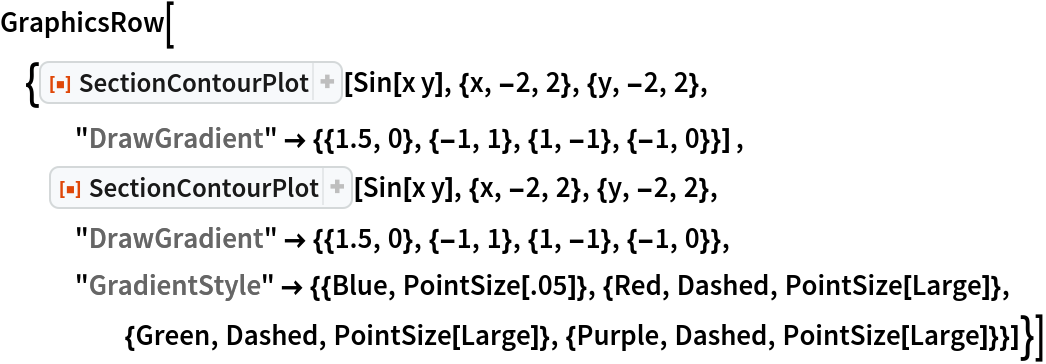Out=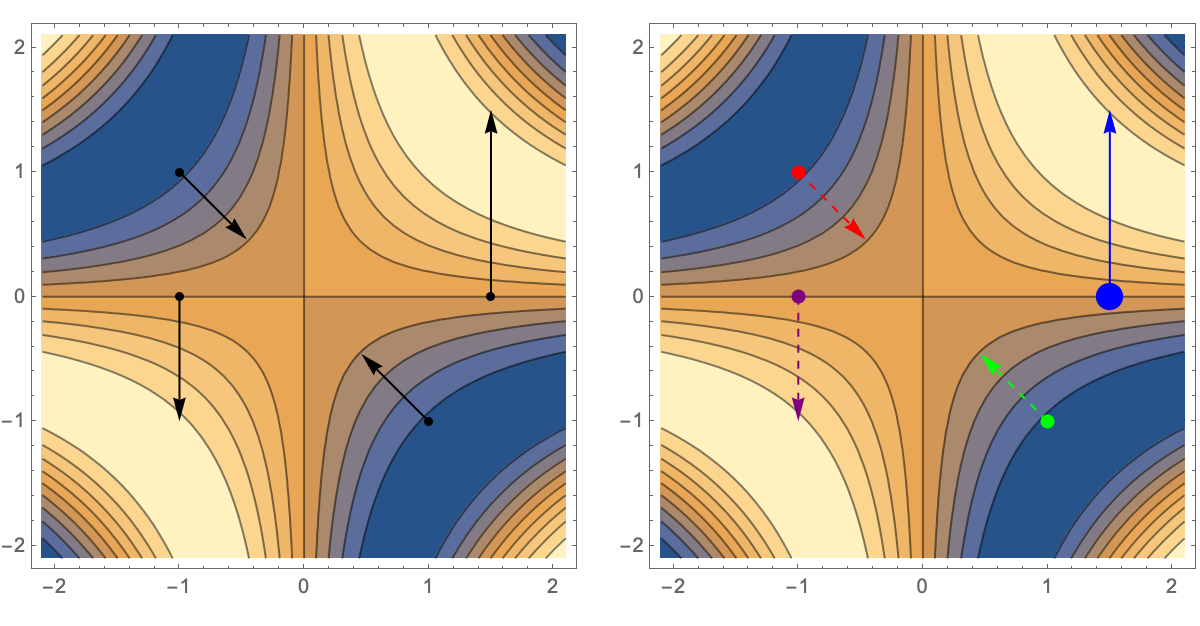#### OSection and OSectionStyle (1)

Oblique sections with a style set for each:

 In:=Out=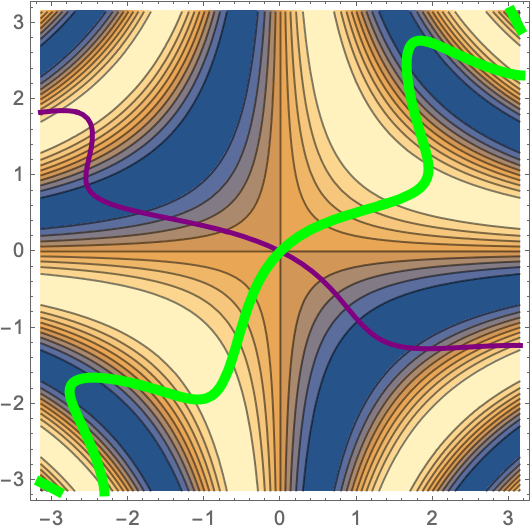#### PlotPointsSection (1)

Setting the number of points used to plot the sections:

 In:=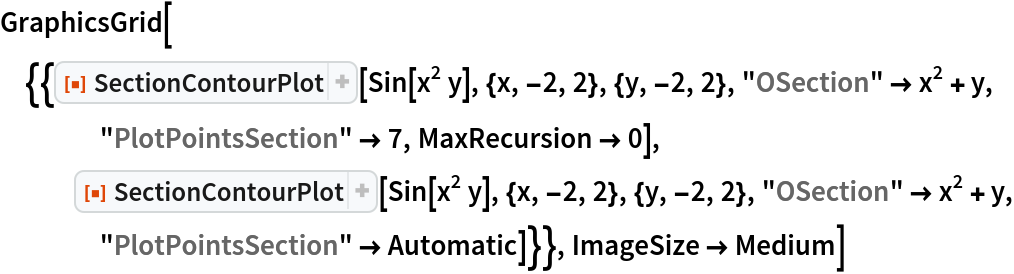Out=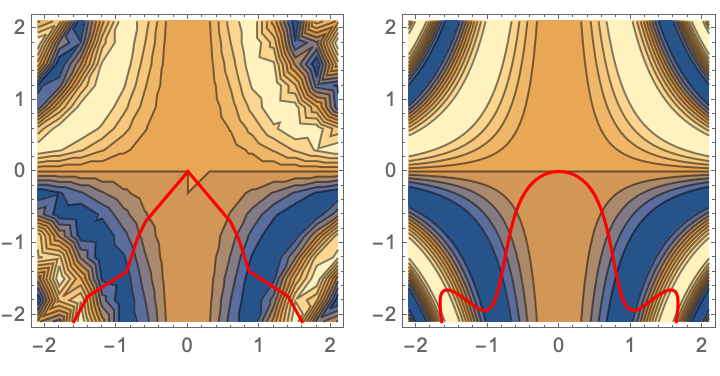#### SectionStyle (1)

Parametrically defined sections with a style set for each:

 In:=Out=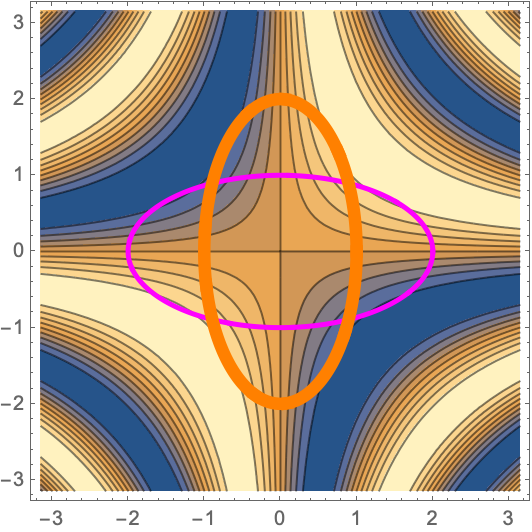#### VSection and VSectionStyle (1)

Vertical sections with a style set for each:

 In:=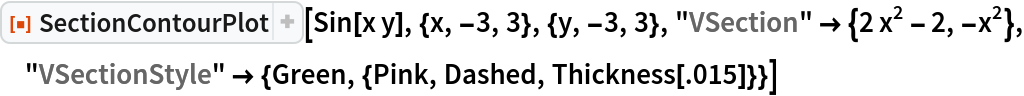Out=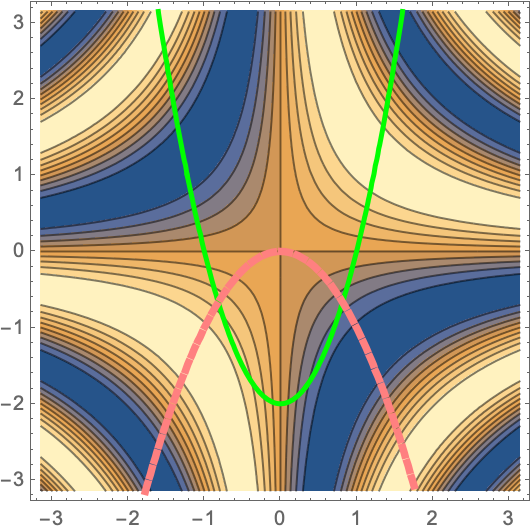#### XSection and XSectionStyle (1)

X sections with a style set for each:

 In:=Out=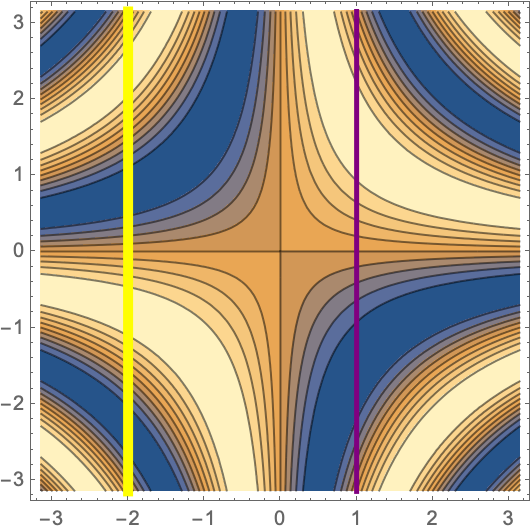#### YSection and YSectionStyle (1)

Y sections with a style set for each:

 In:=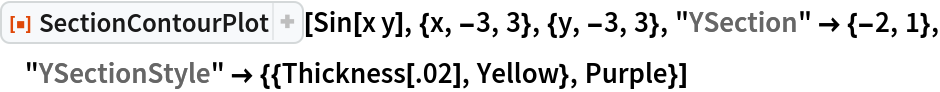Out=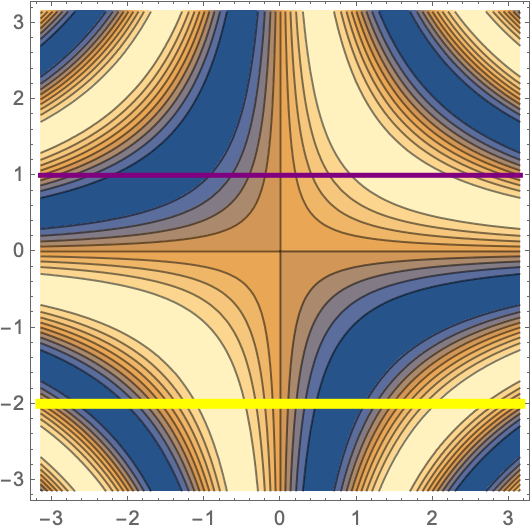#### YSection and YSectionStyle (1)

Z sections with a style set for each:

 In:=Out=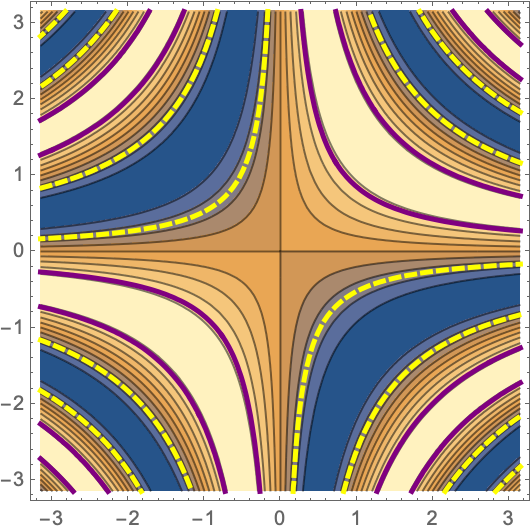## Publisher

Dennis M Schneider

## Version History

• 1.0.0 – 05 November 2019